# Right triangle - leg

Calculate to the nearest tenth cm length of leg in right-angled triangle with hypotenuse length 9 cm and 7 cm long leg.

x =  5.66 cm

### Step-by-step explanation: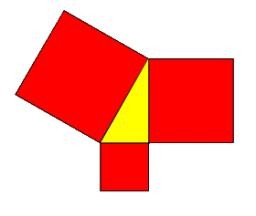Did you find an error or inaccuracy? Feel free to write us. Thank you!Tips to related online calculators
Line slope calculator is helpful for basic calculations in analytic geometry. The coordinates of two points in the plane calculate slope, normal and parametric line equation(s), slope, directional angle, direction vector, the length of the segment, intersections of the coordinate axes, etc.
Pythagorean theorem is the base for the right triangle calculator.

#### You need to know the following knowledge to solve this word math problem:

We encourage you to watch this tutorial video on this math problem:

## Related math problems and questions:

• HypotenuseCalculate the length of the hypotenuse of a right triangle with a catheti 71 cm and 49 cm long.
• HypotenuseCalculate the length of the hypotenuse of a right triangle if the length of one leg is 4 cm and its content area is 16 square centimeters.
• Right trapezoidThe right trapezoid has bases 3.2 cm and 62 mm long. The shorter leg has a length of 0.25 dm. Calculate the lengths of the diagonals and the second leg.
• PrismRight-angled prism, whose base is a right triangle with leg a = 3 cm and hypotenuse c = 13 cm, has the same volume as a cube with an edge length of 3 dm. a) Find the height of the prism b) Calculate the surface of the prism c) What percentage of the cube'
• Sides of right angled triangleOne leg is 1 m shorter than the hypotenuse, and the second leg is 2 m shorter than the hypotenuse. Find the lengths of all sides of the right-angled triangle.
• Rectangular triangle PQRIn the rectangular triangle PQR, the PQ leg is divided by the X point into two segments of which longer is 25cm long. The second leg PR has a length 16 cm. The length of the RX is 20 cm. Calculate the length p of side RQ. The result is round to 2 decimal
• Is right?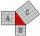Determine whether the triangle with legs (catheti) 19.5 cm and 26 cm and length of the hypotenuse 32.5 cm is rectangular?
• Triangle KLMIn the rectangular triangle KLM, where is hypotenuse m (sketch it!). Find the length of the leg k and the height of triangle h if the hypotenuse's segments are known MK = 5cm and ml = 15 cm.
• Triangle eqCalculate accurate to hundredths cm height of an equilateral triangle with a side length 12 cm. Calculate also its perimeter and content area.
• ArmCalculate the length of the arm r of isosceles triangle ABC, with base |AB| = 14 cm and a height v=18 cm.
• RT perimeterThe leg of the rectangular triangle is 7 cm shorter than the second leg and 8 cm shorter than the hypotenuse. Calculate the triangle circumference.
• Right ΔA right triangle has the length of one leg 11 cm and the hypotenuse 61 cm size. Calculate the height of the triangle.
• Right angled triangleThe hypotenuse of a right triangle is 17 cm long. When we decrease the length of legs by 3 cm, then decrease its hypotenuse by 4 cm. Find the size of its legs.
• SquarePoints A[9,9] and B[-4,1] are adjacent vertices of the square ABCD. Calculate the area of the square ABCD.
• ABC isosceles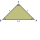ABC isosceles rights triangle the length or each leg is 1 unit what is the length of the hypotenuse AB in the exact form
• OPT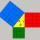What is the perimeter of a right triangle with the legs 14 cm and 21 cm long?
• Diagonal - simple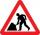Calculate the length of the diagonal of a rectangle with dimensions 5 cm and 12 cm.